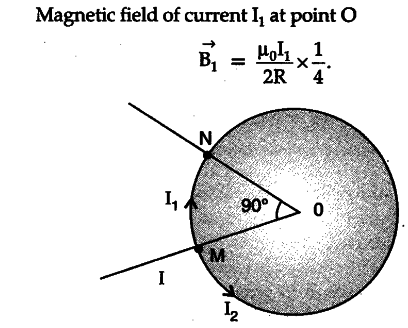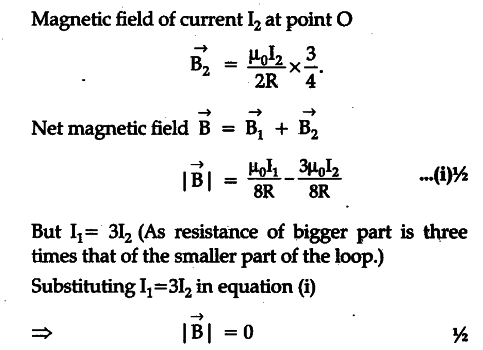# Use Biot-Savart law to derive the expression for the magnetic field due to a circular coil

(a) Use Biot-Savart law to derive the expression for the magnetic field due to a circular coil of radius R having N turns at a point on the axis at a distance V from its centre.
Draw the magnetic field lines due to this coil. (b)A current T enters a uniform circular loop of radius ‘R’ at point M and flows out at N as shown in the figure.
(b)Obtain the net magnetic field at the centre of the loop.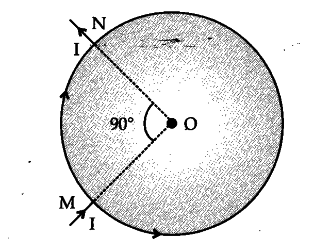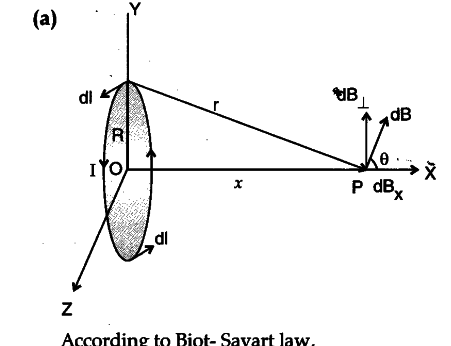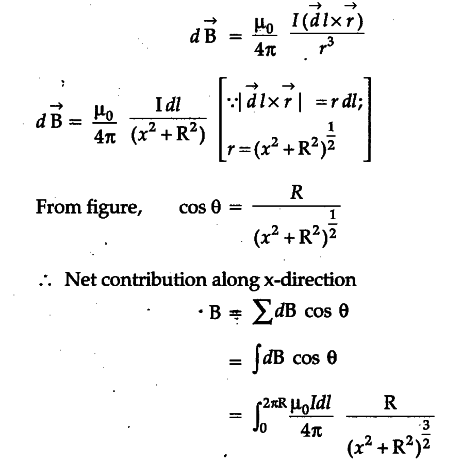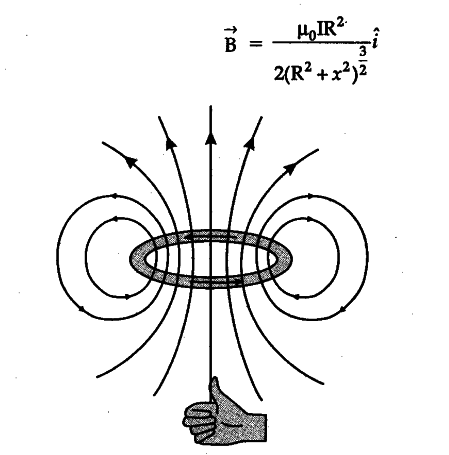(b) Let current I be divided at point M into two parts Ij and I2 ; in bigger and smaller parts of the loop respectively.